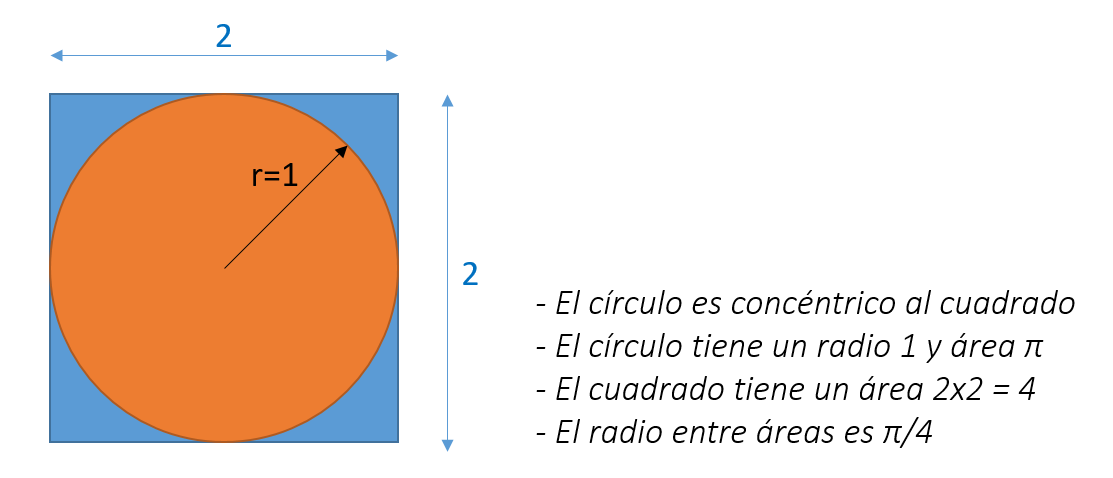# Advanced RAM Analysis Methods: Monte Carlo Simulation

15/10/2021

### Introduction

For complex systems used in the railway, space and aeronautical industry, basic analysis methods (FMECA, RBD, FTA, etc.) of reliability, availability and maintainability (RAM) and subsequent decision-making may not be sufficient in some cases. .

In this chapter we present one of the most used advanced techniques for modeling complex systems: the MONTE CARLO SIMULATION.

The actual development of Monte Carlo methods was done in the realm of the famous Manhattan Project during World War II and research for the development of nuclear weapons. In particular, these probabilistic methods were used to solve certain types of differential equations.

Complex systems are characterized by having a high number of system states, non-constant repair rates and failures (component aging), high dependency on the state of its components, complex maintenance strategies (spare parts, priority, limited resources), etc. Faced with these systems, analytical approaches become impractical:

• Approximate representations that do not fit the real system,
• Excessive time for the development of the analytical model,
• Even total infeasibility of analysis.

Faced with these situations, one of the strategies for RAM analysis is Monte Carlo simulation as a tool for the analysis of complex systems, due to its ability to achieve greater adherence to reality. Monte Carlo Simulation can help us successfully overcome our RAM analysis challenges.

In simplified form, Monte Carlo simulation is a stochastic simulation technique in which multiple independent results are obtained from a modeled system by repeatedly solving the model for randomly sampled (input) values ​​of the input variables and events. The sample of results thus obtained is statistically treated to calculate the result that the system generates.

In other words, applying Monte Carlo involves iteratively executing "numerical experiments" to observe what happens on a mean or average basis, in a large number of executions of a stochastic model.

In the context of RAM, Monte Carlo simulation is used in many applications, for example, to evaluate fault trees (FTA) or Markov models where the failure times of individual components or, the times that the system remains in a Given state follow arbitrary distributions, with the aim of finding the mean operating time between failures or the probability of failure within a given time interval.

In addition, Monte Carlo simulation is used to estimate cumulative density functions in risk assessment, for example, when the average availability within the warranty period is critical and a value must be estimated that is only exceeded with a certain probability. Finally, Monte Carlo simulation is a flexible methodology that can be applied when changes in the state of a system occur in discrete instants of time, which allows modeling complex operation and maintenance scenarios.A key aspect of Monte Carlo simulation is the generation of possible random values ​​of the inputs to the system, which are injected into the model to produce samples of the results. The inputs play a very important role in the quality of the results since the precision of the solution depends on the number of simulations that can be performed and the diversity of the inputs inserted in the model.

That is, more strictly, we can state that mathematical theory establishes that the error of a Monte Carlo estimation technique should decrease proportionally to the square root of the number of attempts.

Practical example: Estimation of the π value with rice grainsThe Monte Carlo method to calculate the number π would work as follows: drawing the square and the circle concentrically, we would start randomly (at random) to throw many grains of rice (one after the other) within the area limited by the square. After this process, we would count the number of rice kernels that fell within the circle (orange zone) of the total number of granites thrown away (the total number of granites would match the orange area granites plus the blue area granites). The ratio of granites of the orange area with respect to the total number of granites would be the same as the ratio of the areas, defined by π / 4. Therefore, the number of granites in the orange area divided by the total number of granites and multiplied by 4, would give us the approximation of π.

The 4 steps to using the Monte Carlo Simulation

• Define the domain of possible inputs. The simulated "universe" must be similar to the universe whose behavior we wish to describe and investigate.
• Generate inputs randomly from a probability distribution over the domain: the inputs must be generated so that their characteristics are similar to the real universe that we are trying to simulate; in particular, the dependencies between the inputs should be represented.
• Calculation of the outputs from the injected inputs. The calculation must be deterministic.
• Add the results to get the output of interest. Typical outputs: histogram, statistical summary (mean, standard deviation ...), confidence intervals, etc.

Clearly, the strengths of Monte Carlo simulation are its flexibility to take into account any distribution of input variables and provide solutions for complex systems that do not lend themselves to analytical solutions, while providing a measure of the precision of the results.

As we have explained in this article, Monte Carlo methods are a simple and convenient alternative that cannot be solved analytically, and this may be the only way to estimate the result of interest. Undoubtedly, these methods are based on the computational speed of computers and their ability to generate pseudo-random numbers from various relevant probability distributions.

In Leedeo Engineering, we are specialists in the development of projects of RAMS, giving support to any level required for tasks RAM and Safety, and both level of infrastructure or equipment on board .Are you interested in our articles about RAMS engineering and Technology?

Sign up for our newsletter and we will keep you informed of the publication of new articles.